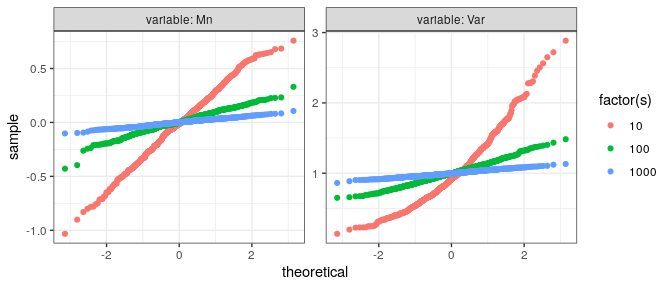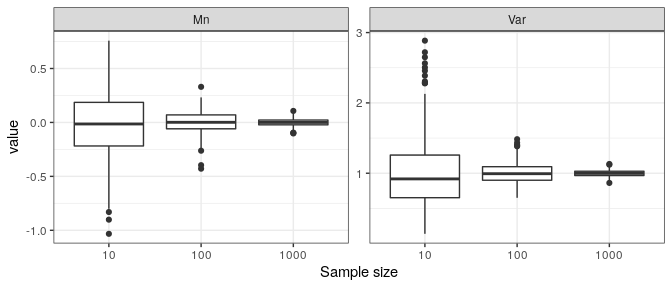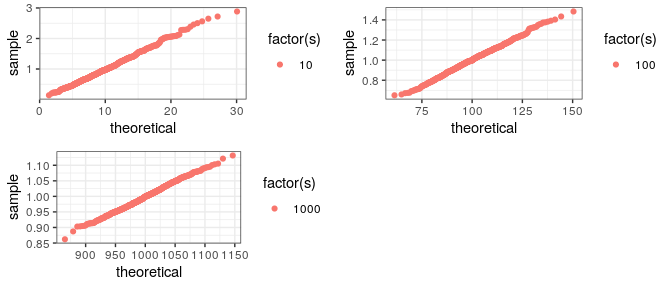# Testing-estimators-with-EstimDiagnostics

library(EstimDiagnostics)
library(doParallel)
library(ggplot2)

registerDoSEQ()
s<-c(1e1,1e2,1e3)
Nmc=6e2

## Diagnostic functions

The main function is Estim_diagnost which takes the simulation and estimation procedure Inference with a sample size as an argument. Inference can return a named vector, a list or a data frame. Estim_diagnost returns a data frame.

Inference<-function(s){
rrr<-rnorm(n=s)
list(Mn=mean(rrr), Var=var(rrr))
}

experiment <- Estim_diagnost(Nmc, s=s, Inference)
#>           Mn       Var  s
#> 1  0.1418157 0.4292025 10
#> 2  0.3447723 0.8160422 10
#> 3 -0.7148383 1.2921048 10
#> 4 -0.3415064 1.0301130 10
#> 5  0.1572961 0.9296052 10
#> 6  0.1819580 1.3757514 10

This data frame consists of columns with estimates (Mn and Var in this case) and a sample size s at which estimates were evaluated.

There are two plot functions that can visualize the results of the simulation study- estims_qqplot and estims_boxplot. The following line plots both estimators from experiment against standard normal distribution. It is known that empirical variance in this case is distributed according to chi-square law. As expected, we see that the distribution of variance converges to a Gaussian law but at small sample sizes notably differs from it.

estims_qqplot(experiment)estims_boxplot(experiment)Each plot has argument sep allowing to switch between plotting different estimators together or separately. If sep=TRUE then the functions return a list of ggplot objects that can be treated and then plotted independently. Here for each plot we set custom distributions qq-plots will be based on:

library(gridExtra)

dist1 <- function(p) stats::qchisq(p, df=1e1)
p1<-estims_qqplot(experiment[experiment$s==1e1,], sep=TRUE, distribution = dist1) dist2 <- function(p) stats::qchisq(p, df=1e2) p2<-estims_qqplot(experiment[experiment$s==1e2,], sep=TRUE, distribution = dist2)

dist3 <- function(p) stats::qchisq(p, df=1e3)
p3<-estims_qqplot(experiment[experiment\$s==1e3,], sep=TRUE, distribution = dist3)

grid.arrange(arrangeGrob(p1[], p2[], p3[], ncol=2))## Unit tests

Once it is shown by means of exploratory analysis that the estimators of interest follow some theoretical distribution, it is desirable to write unit tests for them. This package provides the following expect_ type functions as an extension of testthat package:

• expect_distfit
• expect_gaussian
• expect_mean_equal

In order to test correctness of the mu estimator, expect_mean_equal is called. It uses t-test to test the hypothesis that the empirical mean is different from a chosen value.

s <- 1e1
set.seed(1)

experiment <- Estim_diagnost(Nmc, s=s, Inference)

sam_m <- experiment[,1]
expect_mean_equal(x=sam_m, mu=0)

For variance estimator we make a unit test based on the fact that the empirical variance follows a chi-square distribution. Tests for matching empirical distributions to parametric ones are implemented in expect_distfit function.

sam_v <- experiment[,2]*10
expect_distfit(sample = sam_v, nulldist=pchisq, df=10)